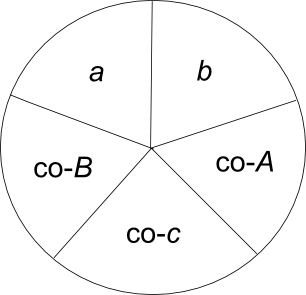# Napier’s mnemonic

John Napier (1550–1617) discovered a way to reduce 10 equations in spherical trig down to 2 equations and to make them easier to remember.

Draw a right triangle on a sphere and label the sides a, b, and c where c is the hypotenuse. Let A be the angle opposite side a, B the angle opposite side b, and C the right angle opposite the hypotenuse c.There are 10 equations relating the sides and angles of the triangle:

sin a = sin A sin c = tan b cot B
sin b = sin B sin c = tan a cot A
cos A = cos a sin B = tan b cot c
cos B = cos b sin A = tan a cot c
cos c = cot A cot B = cos a cos b

Here’s how Napier reduced these equations to a more memorable form. Arrange the parts of the triangle in a circle as below.Then Napier has two rules:

1. The sine of a part is equal to the product of the tangents of the two adjacent parts.
2. The sine of a part is equal to the product of the cosines of the two opposite parts.

For example, if we start with a, the first rule says sin a = cot B tan b. (The tangent of the complementary angle to B is the cotangent of B.) Similarly, the second rule says that sin a = sin c sin A. (The cosine of the complementary angle is just the sine.)

For a more algebraic take on Napier’s rules, write the parts of the triangle as

(p1, p2, p3, p4, p5) = (a , b, co-A, co-c, co-B).

Then the equations above can be reduced to

sin pi = tan pi-1 tan pi+1 = cos pi+2 cos pi+3

where the addition and subtraction in the subscripts is carried out mod 5. This is just using subscripts to describe the adjacent and opposite parts in Napier’s diagram.

Source: Heavenly Mathematics

## 3 thoughts on “Napier’s mnemonic”

1. Eric O. LEBIGOT (EOL)

What is sin a? is it the sine of angle a as seen from the sphere center?

2. Eric: Yes.

3. Thony C

Heavenly Mathematics is a wonderful book